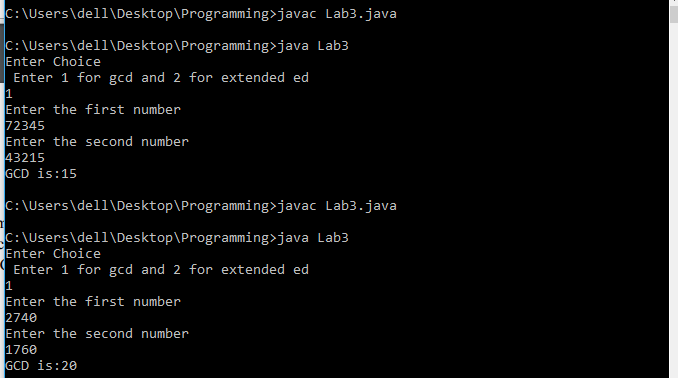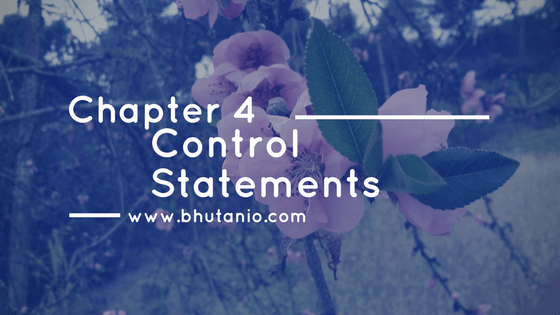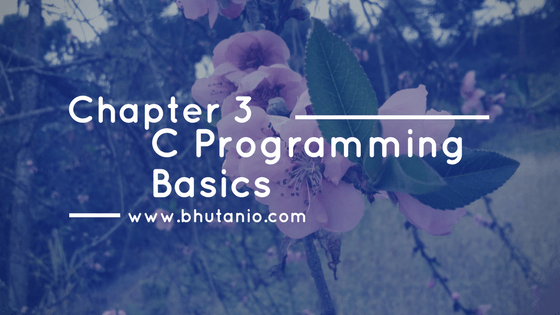# Programming

Programming is the process of writing computer understandable instructions. These instructions can be written using languages known as computer programming languages. Java, Python, C#, C, and C++ are some of the popular ones.

## Implement the Pseudo-codes of Euclid’s AlgorithmLet us look into how to implement Euclid’s algorithm and Euclid’s Extended Algorithms using Java Programming Language. Euclid’s Algorithm Pseudocode Euclid’s Extended Algorithm Pseudocode Question Implement the pseudo-codes of Euclid’s algorithm with recursive function and extended Euclid’s algorithm in any programming language you are comfortable with. Your program should take two integers A and B …

## C Program to Find GCD

C Program to Find GCD: This post discusses how to write C program to find GCD. There are many approaches to find the greatest common divisor (GCD) of any two numbers. It is the highest number that can divide both the numbers. The commonly used method to find the GCD of any two numbers is …

## C Program to Print Fibonacci Numbers

Before looking into the program to print Fibonacci numbers, let us look into what really it is.  Fibonacci numbers are a sequence of numbers known as a Fibonacci sequence which is characterized by the fact that ‘every number after the first two is the sum of the two preceding ones’ as shown below: 1, 1, 2, 3, 5, 8, 13, …

## Structures and Unions in C ProgrammingStructures In the previous chapter, Arrays we have found that arrays are being used to store data of the same type. But often we come across the collection of that of different types that can be considered a unit. For example, in the register book that contains the employee details working in a company, the …

## Arrays in C ProgrammingAn array is an identifier that stores a set of similar data. In another way, arrays can be defined as a data structure that can store a fixed size data in a sequential order where the data in it are of the same type. While arrays can store multiple values, a variable can store only …

## Functions in C ProgrammingA function is a block of code or a group of instructions that as a unit performs a specific task. So functions are programs, and they can be either library functions or programmer written functions. In the programs that we wrote in the previous sections, we have used functions like printf() and scanf(). These functions …

## Control Statements in C ProgrammingControl statements in C: While solving computational problems we come over conditions where we need to deal with the situations that have two possibilities like a thing would happen if other doesn’t happen. Sometimes we may have to keep the program executing repeatedly unless a certain point is being reached. To overcome these situations we …

## C Programming BasicsIn the last chapters, we have looked into some of the import terms in C programming and setting up the environment for writing C programs. In this section, we will be looking into how to write basic C programs like printing texts on the screen. The basics in C programming includes understanding the basic structure …